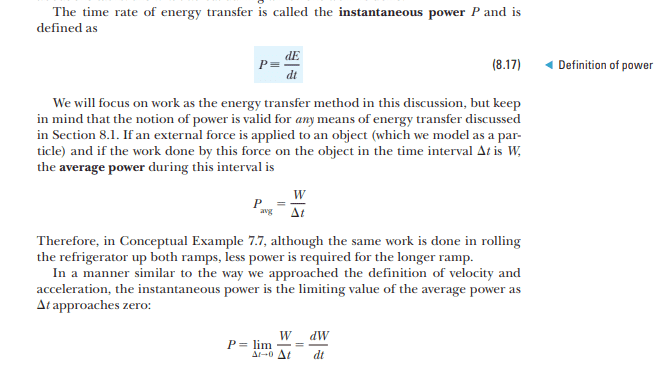# Why do some physics textbooks say P = dK/dt and others say P = dE/dt?

Callumnc1
Homework Statement:
I am interested in why some physics textbooks say P = dK/dt (namely morins classical mechanics) while others say that P = dE/dt (namely physics for scientists and engineers with modern physics)
Relevant Equations:
P = dK/dt = dE/dt
Are they assuming that E = K in physics for scientists and engineers with modern physics, but I though E = K + U?

Many thanks!

Last edited:

Homework Helper
Gold Member
2022 Award
What is P, K and E?

Is P power, is K kinetic energy and E is energy, what kind of energy?

•Callumnc1
Callumnc1
What is P, K and E?

Is P power, is K kinetic energy and E is energy, what kind of energy?
Thanks for your reply @malawi_glenn! Here I'll send you a screen shot what it says.

Callumnc1Homework Helper
Gold Member
2022 Award
The text pretty much explains it.

The generic definition of power is ANY energy transfer, like transfer of heat etc.

Then in the case of an external force that is performing work, you will get P = dW/dt

•Callumnc1
Callumnc1
The text pretty much explains it.

The generic definition of power is ANY energy transfer, like transfer of heat etc.

Then in the case of an external force that is performing work, you will get P = dW/dt
Ok thank you @malawi_glenn!